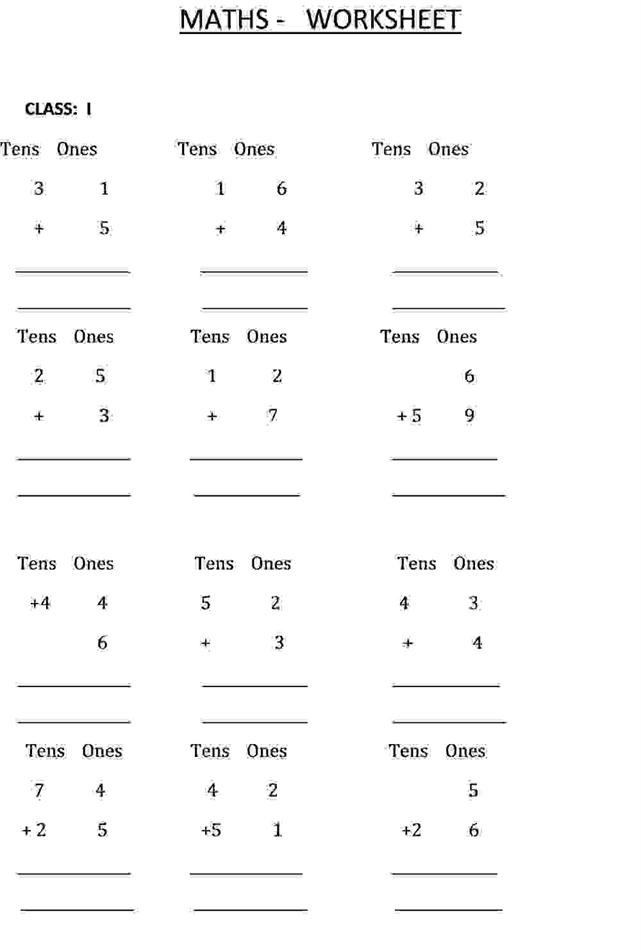Previous

# Addition calculation - Class 1 Maths Worksheet

Posted Date: 21 Feb 2012     Posted by: Hafeez
Resources » Addition calculation - Class 1 Maths WorksheetOpen PDF file and PrintGet Addition calculation - Class 1 Maths Worksheet

Find more: Addition calculation Class 1 Maths Worksheet   Addition calculation sum   Class 1 Maths Worksheet page